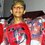# Irrational Math…

No number is equal to any other no. except itself Stupid statement, isn't it?? Well, you might think that I declare it as stupid, and it is accepted worldwide. But here… Take a rational x and its negative,i.e., -x. Square them, and they becomex^{2}. Now, They are equal. Hence, taking square root on both sides, we get: X=-X Hence, disprovedNote by Vikram Venkat
6 years, 7 months ago

This discussion board is a place to discuss our Daily Challenges and the math and science related to those challenges. Explanations are more than just a solution — they should explain the steps and thinking strategies that you used to obtain the solution. Comments should further the discussion of math and science.

When posting on Brilliant:

• Use the emojis to react to an explanation, whether you're congratulating a job well done , or just really confused .
• Ask specific questions about the challenge or the steps in somebody's explanation. Well-posed questions can add a lot to the discussion, but posting "I don't understand!" doesn't help anyone.
• Try to contribute something new to the discussion, whether it is an extension, generalization or other idea related to the challenge.

MarkdownAppears as
*italics* or _italics_ italics
**bold** or __bold__ bold
- bulleted- list
• bulleted
• list
1. numbered2. list
1. numbered
2. list
Note: you must add a full line of space before and after lists for them to show up correctly
paragraph 1paragraph 2

paragraph 1

paragraph 2

[example link](https://brilliant.org)example link
> This is a quote
This is a quote
    # I indented these lines
# 4 spaces, and now they show
# up as a code block.

print "hello world"
# I indented these lines
# 4 spaces, and now they show
# up as a code block.

print "hello world"
MathAppears as
Remember to wrap math in $$ ... $$ or $ ... $ to ensure proper formatting.
2 \times 3 $2 \times 3$
2^{34} $2^{34}$
a_{i-1} $a_{i-1}$
\frac{2}{3} $\frac{2}{3}$
\sqrt{2} $\sqrt{2}$
\sum_{i=1}^3 $\sum_{i=1}^3$
\sin \theta $\sin \theta$
\boxed{123} $\boxed{123}$

Sort by:

u should go a bit deeper inside ur proof .....for a correct mathematical proof i think counts in it all the possible perspstives .....:-)

- 6 years, 6 months ago

Well, the proof is never the basis. I am waiting for a counter-statement

- 6 years, 5 months ago

Just look here my friend

Let's oversimplify it ,

x^2=x^2 1st condition ,

xx=xx

2nd condition,

(-x) *(-x) = x * x

Now , you divide the whole equation by x, you see

x=x , you can only remove x from the equation not ( -1)*(-1)

That's why I said you should consider all possible perspectives ..!!

- 6 years, 5 months ago

There is no such number where its predecessor or its successor is the negative value of itself, just looking at the number 1, it has a predecessor of 0, and a successor of 2, neither equaling -1.

- 6 years, 7 months ago

Have a good look at it bro!!!

- 6 years, 7 months ago

be careful with squares and square roots , think once again for a while

- 6 years, 7 months ago

@U Z What happened @megh choksi ???

- 6 years, 5 months ago